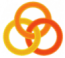General
 Course Home Page ESSLLI 2004Material
Lecture 1
 Slides Practical Session
Lecture 2
 Slides Practical Session
Lecture 3
 Slides Practical Session
Lecture 4
 Slides Practical Session
Lecture 5
 Slides Practical Session

# Accumulators

Compare the following two definitions of the `list-length` predicate. Do a trace to see how the result is computed.

```list_length1([],0).
list_length1([_|Tail],Length) :-
list_length1(Tail,TailLength),
Length is TailLength + 1.
```
```list_length2(List,Length) :- list_length2(List,0,Length).

list_length2([],Length,Length).
list_length2([_|Tail],Accumulator,Length) :-
NewAcc is Accumulator + 1,
list_length2(Tail,NewAcc,Length).
```

The main difference that you can observe when doing a trace is that `list_length1` computes the result when coming out of the recursion, while `list_length2` computes the result while going into the recursion. At the time where the bottom of the recursion (the empty list) has been reached, the length of the list has already been established. To be able to do this, `list_length2` uses an accumulator, i.e., an argument for storing intermediate results. In the beginning, the accumulator is instantiated with 0. In each recursive call, the accumulator argument is increased by one (to indicate that one more list element has been processed). By the time the empty list is reached, the accumulator argument contains a number corresponding to the length of the list.

The accumulator adds an extra argument to the predicate which has to be instantiated when the predicate is first called. We use a wrapper predicate to do this automatically:

```list_length2(List,Length) :- list_length2(List,0,Length).
```

Define an accumulator version of the predicate `max/2` that you had to define in this exercise.
Hint

Define a predicate `mirror(+InList,?OutList)` which takes a list as argument and turns it around. For example, the query `mirror([a,b,c,d],L)` should yield the answer `L = [d,c,b,a]`. Use an accumulator and don't use `append`, such that the result is ready when the empty list is reached.
Hint

Define an accumulator version of the flattening predicate defined in this exercise.
Hint